# Resolvent

(diff) ← Older revision | Latest revision (diff) | Newer revision → (diff)

A resolvent of an algebraic equation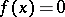of degreeis an algebraic equation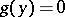, with coefficients that rationally depend on the coefficients of, such that, if the roots of this equation are known, the roots of the given equationcan be found by solving simpler equations of degrees not exceeding. A rational expression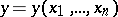itself is sometimes called a resolvent.

Letbe a separable polynomial over a fieldwith Galois group, and letbe a normal subgroup of. Letbe a rational expression inthat remains invariant under all permutations of the rootsbelonging to, and let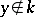. Thenis a root of some equationwith coefficients from, the Galois group of which is a proper quotient group of. Thus, solving the equationreduces to solving the equationand solving the equationover the field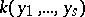.

For example, in order to solve an equation of degree: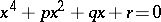(every equation of degreeis reducible to this form), the following cubic resolvent is used: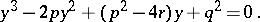Its roots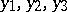are related to the rootsby the relations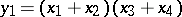,,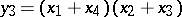. The rootsare determined by the Cardano formula, which also makes it possible to determine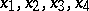.

Successive application of the resolvent method permits one to solve any equation with a solvable Galois group by reduction to solving a chain of equations with cyclic Galois groups. Lagrange's resolvent is used in solving the latter.

Letbe an equation over a fieldwith a cyclic Galois groupof order, and letcontain a primitive-th root of unity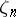. For an elementwhich belongs to the splitting field of the polynomial(cf. Splitting field of a polynomial), and for a characterfrominto the group of-th roots of unity, Lagrange's resolvent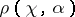is defined by the formula: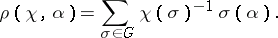(*)

Let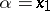be one of the roots of the polynomialand letrun through the characters of. Then for the system of linear equations (*) the rootscan be determined if the Lagrange resolvents are known for all charactersof.

Forthe relationis fulfilled, showing that the elements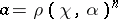and, for any integer, are invariant underand are therefore uniquely defined rational expressions in the coefficients of the polynomialand the root. Ifgenerates the group of characters of, then the following equalities hold: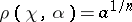and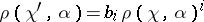for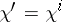.

Any algebraic equation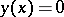that is irreducible over a given field (see Galois theory) and that is such that as a result of the adjunction of one of its roots to this field a field is obtained that contains all roots of the equation, is called a Galois resolvent of.

How to Cite This Entry:
Resolvent. Encyclopedia of Mathematics. URL: http://encyclopediaofmath.org/index.php?title=Resolvent&oldid=12785
This article was adapted from an original article by V.I. Sobolev (originator), which appeared in Encyclopedia of Mathematics - ISBN 1402006098. See original article